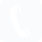2013年9月全国计算机等级《二级VB》上机临考冲刺试卷(2)
1、【 单选题

2、【 单选题

Visual Basic 只能通过过程调用执行通用过程

Sub过程和Function过程都有返回值

3、【 单选题

4、【 单选题

Sub S(X As Integer，y As Integer)
Static z As Integer
y=x*x+Z
z=y
End Sub
Private Sub Command1 Click（　　）
Dim i As Integer．z As Integer
m=0
z=0
For i=1 T0 3
S i，Z
m=m+z
Next i
Label1．Caption=Str(m)

50
20
14
7

5、【 单选题

6、【 单选题

a\$="visual Basic Programming"
b\$="C++"
C\$=UCase(Left\$(a\$，7))＆b\$＆Right\$(a\$，1 2)后，变量c\$的值为（　）。 [1分]
Visual BASIC Programming
VISUAL C++Programming
Visual C++Programming
VISUAL BASIC Programming

7、【 单选题

Private Sub Command1 Click（　　）
Dim n As Long
Dim flag As Boolean
n=InputBox("输入一个正整数")
S=Trim(Str(n))
For i=2 To Len(s)
If Mid(s，i-1，1)
Next i
If i=Len(S)Then flag=True Else flag=False
If flag Then
Print n；"是降序数"
Else
Print n；"不是降序数"
End If
End Sub

If i=Len(s)+1 Then flag=False Else flag=True
If i=Len(s)+1 Then flag=True Else flag=False
If i=Len(s)-1 Then flag=False Else flag=True
If i=Len(s)-1 Then flag=True Else flag=False

8、【 单选题

9、【 单选题

10、【 单选题

Private Sub Form Click（　　）
Dim n As Integcr
x=0
n=InputBox("请输入一个整数")
For i=1 To i
for j =1 To j
x=x+1
Next j
Next i
Print x
End sub

13
14
15
16

11、【 单选题

Dim ss As String
Private Sub Text1 KeyPress(KeyAscii As Integer)
If Chr(KeyAscii)<>""Then ss=ss+Chr(KeyAscii)
End Sub
Private Sub Command1 Click（　　）
Dim m As String，i As Integer
For i=Len(ss)To 1 Step-1
m=m+Mid(SS，i，1)
Next
Text1．Text=UCase(m)
End Sub

NUMtBER l00
REBMUN
REBM UN l00
001 REBMUN

12、【 单选题

Private Sub Timer1 Timer（　　）
Static flag As Integer
If Flag=0 Then Flag=1
Flag=-flag
If flag=1 Then
Text1．ForeColor=＆HFF＆ ＆HFF＆为红色
Else
Text1．ForeColor=＆HCOO＆ ＆HCOO＆为绿色
End If
End Sub

flag的值只可能取0或1

13、【 单选题

14、【 单选题

Forml．frm

TheFirst．frm
Form1．vbp

15、【 单选题

Dim a，b，C，d As Single
Dim X As Single
f1=10
b=30
c=400
If b>fl Then
d=fl：fl=b：b=d
End If
If b>C Then
X=b
Else If fl>c Then
X=c
Else
X=a
End If [1分]
10
30
400
430

16、【 单选题

Dim a(3，5)As Integer For i=1 To 3
For j=1 To 5
a(i，j)=i+j
Print a(i，j)；
Next
Print
NextFor i=1 To 5
For j=1 To 3
Print a(j，i)；
Next
Print
Next
For i=1 T0 3
For j=1 To 5
Print a(j，i)；
Next
Print Next
For j=l To 5
For i=1 To 3
Print a(j，i)；
Next
Print
Next
For i=1 To 5
For=1 To 3
Print a(i，j)：
Next
Print
Next

17、【 单选题

Private Sub Cornmand1 Click（　　）
P1=1
Sign=1
n=20000
For k=3 To r
Sign=-Sign
PI=PI+SiRn／k
Next k
Print PI*4
End Sub

18、【 单选题19、【 单选题

20、【 单选题

Type Books
Name As String*10
TelNum As String*20
End，Type

Private Sub Command1 Click（　）
Dim B AS Books
Open"Person txt"For Output As#1
Name=InputBox(”输入姓名”)
relNum=lnputBox(”输入电话号码”)
Wlite#1 B Name，B TelNum
Ch,se#1
End Sub
Private Sub Command1 Click（　）
Dim B AS Books
Open"Person txt"For Input As#1
>Name=InputBox("输入姓名")
TelNum=InputBox("输入电话号码")
Print#1，B．Name，B．TelNam
Close#1
End Sub
Private Sub Command1 Click（　）
Dim BAS Books
Open"Person txt"For Output As#1
Name=InputBox("输入姓名")
TelNum=InputBox("输入电话号码")
Write#1，B
Close#1
End Sub
Private Sub Commandl Click（　）
Open"Person txt"For Input As#1
Name=lnputBox("输入姓名")
TelNum=lnputBox("输入电话号码")
Prim#1 Name TelNum
Close#1
End Sub

21、【 单选题

Dir1．Path=Drive1．Path
File1．Path=Dri1．Path
File1．Path=Drive1．Path
Drive1．Drive=Dri1．Path

22、【 单选题

Private Sub Click—MouseDown(Button As Integer，Shift As Integer，X As Single，Y
As Single)
Print"VB Program"
End Sub [1分]

23、【 单选题

FontUnderline
FontBold
FontSlope
FontItalic

24、【 单选题

Private Sub Text1_KeyPress(KeyAscii As Integer)
Dim str As String，n As Integer
str=UCase(Chr(KeyAscii))
n=Len(str)。
Text1．Text=String(n，str)
End Sub

A.pp
pP
ｐｐ
Pp

25、【 单选题

Form1 MouseDown
Win MouseDown
Form MouseDown
MouseDown_Form1

26、【 单选题

27、【 单选题

Option Base 0
Private Sub Command1 Click（　）
Dim A1(4)As Integer，A2(4)As Integer
For k=0 To 2
A1(k+1)=InputBox(”请输入一个整数”)
A2(3-k)=A1(k+1)
Next k
Print A2(k)
End Sub

0
1
2
3

28、【 单选题

Lis tBox
ComboBox
TextBox
Label

29、【 单选题

30、【 单选题

If（　　）ption1．Value—True Then

If Option1．Value Then
If Option1一True Then
If Value=True Then
If Option1 Then

31、【 单选题

Private Sub Command1_Click（　　）
CommonDialog．DefaultExt="doc"
CommonDialog．FileName="VB．txt"
CommonDialog．Filter="All(*．*)|*．*|Word|．Doc|"
CommonDialog．FilterIndex=1
CommonDialog．ShowSave
End Sub

DefaultEx1属性与FileName属性所指明的文件类型不一致，程序出错

32、【 单选题

1或多于1
0或1

33、【 单选题

Data控件
Form控件
CommonDialog控件
VBComboBox控件

34、【 单选题

35、【 单选题

Private Sub Command1 Click（　　）
Dim n
If Text1．Text<>"123456"Then
n=n+1
Print"口令输入错误”＆n＆”次"
End If
End Sub36、【 单选题

10
20
30
200

37、【 单选题[1分]
Private Sub Vorm Click（　　）
End Sub
Private Sub Command1 Click（　　）
End Sub
Private Sub Form Click（　　）
End Sub
Private Sub Form Click（　　）
End Sub

38、【 简答题39、【 简答题根据题意，新建“标准EXE”工程，在窗体上添加一个Labe1控件和两个Option控件，设置Label1的名称为Label1、Caption属性为空，Option的名称分别为0ption1和Option2、Caption属性分别为“教授”和“研究员”。设计完成后，进入代码窗口编写如下代码：40、【 简答题2页，共41个题库
2页，共41个题库

400-886-8169

©2022 轻速云 苏ICP备16049646号-1 轻速云科技提供专业的在线考试系统、在线培训系统客服热线:400-886-8169 | 周一至周日 8:00-22:00